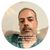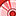Announcements
Applications are open for the 2024 Qlik Luminary Program. Apply by December 15 here.
cancel
Showing results for
Did you mean:Partner - Contributor II

## aggr() condition in set analysis

Hi

I am using aggr() function to calculate a condition a define a dimension:

if(aggr (avg (COMPLETE), CDC_COMPL)=1,'ok','not ok')

Now I need to sum(SALES) only for the CDC where the condition above is ok.

for example I tried:

sum({<CDC=(if(aggr (avg (COMPLETE), CDC_COMPL)=1,CDC_COMPL))>} SALES)

however the sintax is returning an error,

Can anyone suggest how this can be done?

Thanks,

Marco

1 Solution

Accepted SolutionsMVP

May be this -

sum({<CDC={"=avg(aggr (avg (COMPLETE), CDC_COMPL))=1"}>} SALES)

4 RepliesSpecialist III

I think it should be this:

sum({<CDC=(if(aggr (avg (COMPLETE), CDC_COMPL)=1,CDC_COMPL,0))>} SALES)

I added the ",0" to complete the If statement.  If the IF test is 'not ok', it will sum zero.MVP

May be this -

sum({<CDC={"=avg(aggr (avg (COMPLETE), CDC_COMPL))=1"}>} SALES)Partner - Contributor II
Author

thanks Digvijay Singh. it works great

can I ask you when and why I need to use double quotes? and why you put an equal sign before the statement inside the quotes?MVP

Glad to hear that it worked for you. Set Analysis broadly has 3-4 different formats which are being utilized based on the kind of filtering we need.  Double quote with equal sign is used to write search string inside the element list(statement inside {}).

When we need to write condition in the element list like {X<=0} and the functions like aggr/sum/max etc then it doesn't need to be enclosed with \$ sign. You may like to see this attached doc for different formats in different situations.Tags
Community Browser